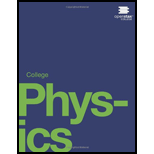# Construct Your Own Problem Consider a space sail such as mentioned in Example 29.5 Construct a problem in which you calculate the light pressure on the sail in N/m 2 produced by reflecting sunlight. Also calculate the force that could be produced and how much effect that would have on a spacecraft. Among the things to be considered are the intensity of sunlight, its average wavelength, the number of photons per square meter this implies, the area of the space sail, and the mass of the system being accelerated.### College Physics

1st Edition
Paul Peter Urone + 1 other
Publisher: OpenStax College
ISBN: 9781938168000### College Physics

1st Edition
Paul Peter Urone + 1 other
Publisher: OpenStax College
ISBN: 9781938168000

#### Solutions

Chapter
Section
Chapter 29, Problem 47PE
Textbook Problem

## Construct Your Own ProblemConsider a space sail such as mentioned in Example 29.5 Construct a problem in which you calculate the light pressure on the sail in N/m2 produced by reflecting sunlight. Also calculate the force that could be produced and how much effect that would have on a spacecraft. Among the things to be considered are the intensity of sunlight, its average wavelength, the number of photons per square meter this implies, the area of the space sail, and the mass of the system being accelerated.

Expert Solution
To determine

The light pressure on the sail produced by the reflecting sunlight and the force exerted on the sail.

### Explanation of Solution

Formula used:

The expression for the force exerted on the sail is given as,

F=PcF=IAc        ...... (1)

The expression for the pressure on the sail is,

Psail=FA       ...... (2)

Calculation:

Consider a sail of area6.0×105m2and mass5.0×103kgis placed in an orbit facing the sun. The intensity of the sunlight is1370W/m2.

Substitute the values in equation (1) to calculate the force.

F=(1370W/m2)×(6.0×105m2)3×108m/sF=2

### Want to see the full answer?

Check out a sample textbook solution.See solution

### Want to see this answer and more?

Bartleby provides explanations to thousands of textbook problems written by our experts, many with advanced degrees!

See solution